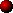OPERATION OF THE TESLA COIL

A Tesla Coil is an air-cored resonant transformer. It has some similarities with a standard transformer but the mode of operation is somewhat different. A standard transformer uses tight coupling between its primary and secondary windings and the voltage transformation ratio is due to turns ratio alone. In contrast, a Tesla Coil uses a relatively loose coupling between primary and secondary, and the majority of the voltage gain is due to resonance rather than the turns ratio. A normal transformer uses an iron core in order to operate at low frequencies, whereas the Tesla Coil is air-cored to operate efficiently at much higher frequencies.

A typical Tesla Coil circuit diagram is shown below.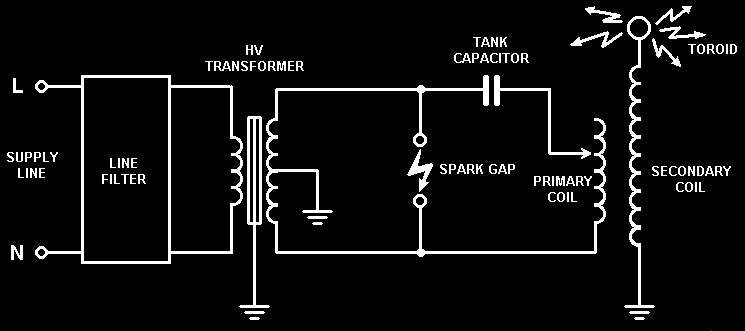The operation of the Tesla Coil is as follows:-

1. The spark gap initially appears as an open-circuit. Current from the HV power supply flows through a ballast inductor and charges the primary tank capacitor to a high voltage. The voltage across the capacitor increases steadily with time as more charge is being stored across its dielectric.
2.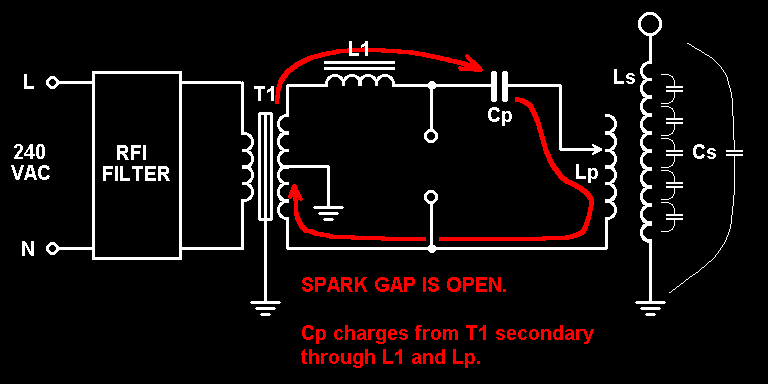3. Eventually the capacitor voltage becomes so high that the air in the spark gap is unable to hold-off the high electric field and breakdown occurs. The resistance of the air in the spark gap drops dramatically and the spark gap becomes a good conductor. The tank capacitor is now connected across the primary winding through the spark gap. This forms a parallel resonant circuit and the capacitor discharges its energy into the primary winding in the form of a damped high frequency oscillation. The natural resonant frequency of this circuit is determined by the values of the primary capacitor and primary winding, and is usually in the low hundreds of killohertz.
4.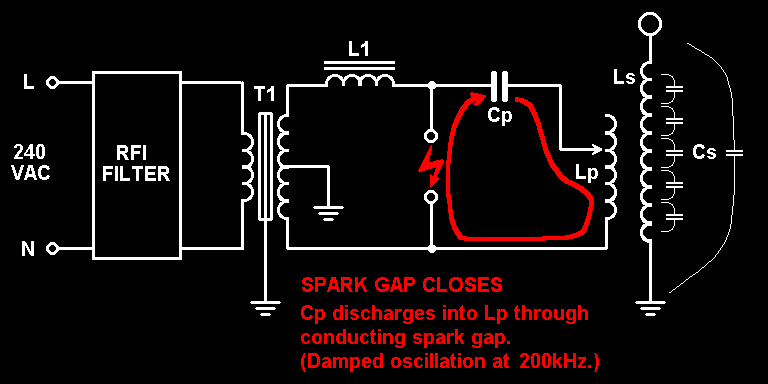During the damped primary oscillation energy passes back and forth between the primary capacitor and the primary inductor. Energy is stored alternately as voltage across the capacitor or current through the inductor. Some of the energy from the capacitor also produces considerable heat and light in the spark gap. Energy dissipated in the spark gap is energy which is lost from the primary tank circuit, and it is this energy loss which causes the primary oscillation to decay relatively quickly with time.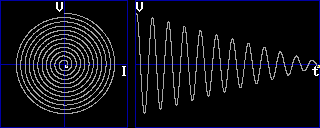I like this spiral diagram because I think it shows how the voltage and current are 90 degrees out of phase. The distance of the dot from the origin represents the amount of energy in the system as the oscillation decays. It also reminds me of a primary coil shape !

5. The close proximity of the primary and secondary windings causes magnetic coupling between them. The high amplitude oscillating current flowing in the primary causes a similar oscillating current to be induced in the nearby secondary coil.

6. The self capacitance of the secondary winding and the capacitance formed between the Toroid and ground result in another parallel resonant circuit being made with the secondary inductance. Its natural resonant frequency is determined by the values of the secondary inductance and its stray capacitances. The resonant frequency of the primary circuit is deliberately chosen to be the same as the resonant frequency of the secondary circuit so that the secondary is excited by the oscillating magnetic field of the primary.
7.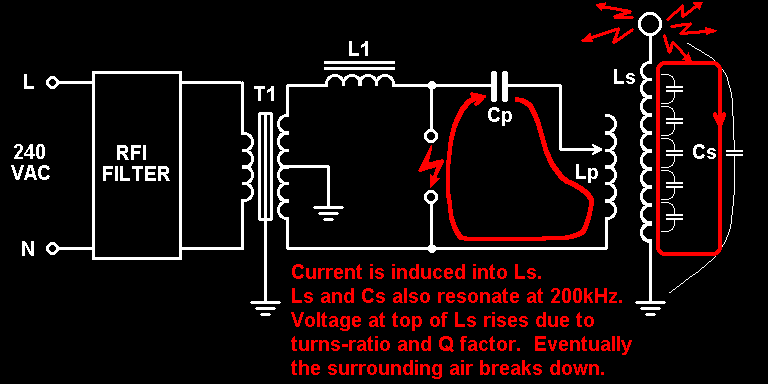8. Energy is gradually transferred from the primary resonant circuit to the secondary resonant circuit. Over several cycles the amplitude of the primary oscillation decreases and the amplitude of the secondary oscillation increases. The decay of the primary oscillation is called "Primary Ringdown" and the start of the secondary oscillation is called "Secondary Ringup". When the secondary voltage becomes high enough, the Toroid is unable to prevent breakout, and sparks are formed as the surrounding air breaks down.
9.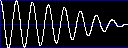### "Primary Ringdown" to first primary notch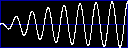### "Secondary Ringup" to first maximum

10. Eventually all of the energy has been transferred to the secondary system and none is left in the primary circuit. This point is known as the "First primary notch" because the amplitude of the primary oscillation has fallen to zero. It is the first notch because the energy transfer process usually does not stop here. In an ideal system the spark gap would cease to conduct at this point, when all of the energy is trapped in the secondary circuit. Unfortunately, this rarely happens in practice.

11. If the spark gap continues to conduct after the first primary notch then energy begins to transfer from the secondary circuit back into the primary circuit. The secondary oscillation decays to zero and the primary amplitude increases again. When all of the energy has been transferred back to the primary circuit, the secondary amplitude drops to zero. This point is known as the "First secondary notch", because there is no energy left in the secondary at this time.
12.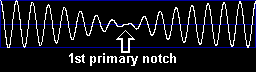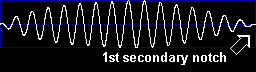13. This energy transfer process can continue for several hundred microseconds. Energy sloshes between the primary and secondary resonant circuits resulting in their amplitudes increasing and decreasing with time. At the instants when all of the energy is in the secondary circuit, there is no energy in the primary system and a "Primary notch" occurs. When all of the energy is in the primary circuit, there is no energy in the secondary and a "Secondary notch" occurs.
14.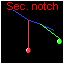In the animation opposite the front pendulum represents the primary voltage and the rear pendulum represents the secondary voltage. Notice how the amplitude of each pendulum changes as energy is transferred back and forth from one to the other. A mechanical model such as this can easily be built and provides a good analogy to the electrical case. It really does work ! The "notches" are clearly visible when one pendulum appears to momentarily stop.

15. Each time energy is transferred from one resonant circuit to the other, some energy is lost in either the primary spark gap, RF radiation or due to the formation of sparks from the secondary. This means that the overall level of energy in the Tesla Coil system decays with time. Therefore both the primary and secondary amplitudes would eventually decay to zero.
16.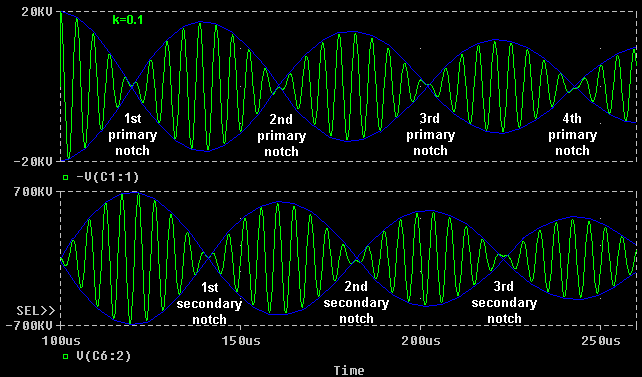17. After several transfers of energy between primary and secondary, the energy in the primary will become sufficiently low that the spark gap will cool. It will now stop conducting at a primary notch when the current is minimal. At this point any remaining energy is trapped in the secondary system, because the primary resonant circuit is effectively "broken" by the spark gap going open-circuit.
18.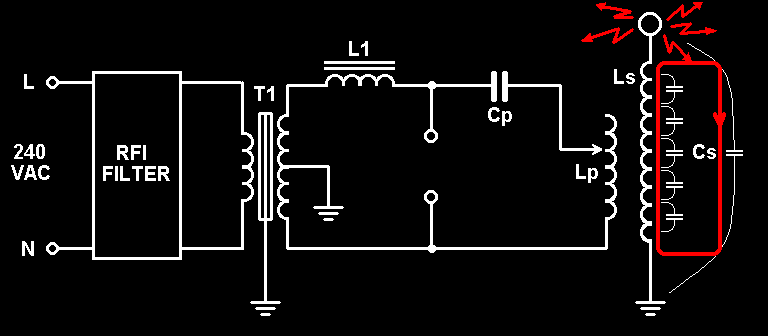19. The energy left in the secondary circuit results in a damped oscillation which decays exponentially due to resistive losses and the energy dissipated in the secondary sparks.
20.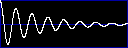### Secondary Ringdown after spark gap stops conducting

21. Since the spark gap is now open-circuit the tank capacitor begins to charge again from the HV supply, and the whole process repeats again.

It should be noted that this repeating process is an important mechanism for the generation of long sparks. This is because successive sparks build on the hot ionised channels formed by previous sparks. This allows sparks to grow in length over several firings of the system. In practice the whole process described above may take place several hundred times per second.

But how does the Tesla Coil produce such a massive secondary voltage ?

Now for the maths bit…

The terrific voltage gain of the Tesla Coil comes from the fact that the energy in the large primary tank capacitor is transferred to the comparatively small stray capacitance of the secondary circuit. The energy stored in the primary capacitor is measured in Joules and is found from the following formula:

Ep = 0.5 Cp Vp²

If, for example, the primary capacitor is 47nF and it is charged to 20kV then the stored energy can be calculated.

Ep = 0.5 x 47n x (20000)² = 9.4 Joules

If we assume there are no losses in the transfer of energy to the secondary winding, the theory of conservation of energy states that this energy will be transferred to the secondary capacitance Cs. Cs is typically around 25pF. If it contains 9.4 Joules of energy when the energy transfer is complete, we can calculate the voltage:

Es = 0.5 x 25p x Vs² = 9.4

Vs² = 9.4 / (0.5 x 25p)

Vs = 867 kV

The theoretical voltage gain of the Tesla Coil is actually equal to the square root of the Capacitance ratio.

Gain = sqrt (Cp / Cs)

The voltage gain can also be calculated in terms of the inductances…

For the Tesla Coil to work, the resonant Frequencies of the Primary circuit and the Secondary circuit must be identical. I.e. Fp must equal Fs.

Fp = 1 / 2 pi sqrt (LpCp) = Fs = 1 / 2 pi sqrt (LsCs)

Therefore: LpCp = LsCs

The ratio of the inductances is the inverse of the ratio of the capacitances, and therefore the voltage gain is as follows:

Gain = sqrt (Ls / Lp)

All of the above equations calculate the theoretical maximum voltage gain. In practice the voltage at the top of the secondary will never get quite this high because of several factors:-

1. The above equations assume that all of the energy from the primary capacitor makes the journey into the secondary capacitor. In practice some energy is lost due to resistance of the windings of both coils.

2. A significant proportion of the initial energy is lost as light, heat, and sound in the primary spark gap.

3. The primary and secondary coils act like antennas and radiate a small amount of energy in the form of radio waves.

4. The formation of corona or arcs from the Toroid to nearby grounded objects ultimately limits the peak secondary voltage.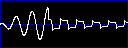The waveform opposite shows how the secondary voltage drops drastically when an arc forms between the toroid and a nearby grounded object. This is an approximate representation of a real waveform observed whilst a running coil discharged to an earthed target 12 inches away. The secondary voltage rises to around 300kV in only 3 cycles, This is sufficient to breakdown the 12 inch gap, and the arc which is formed loads the secondary reducing the voltage.

The size of the Toroid (or discharge terminal) is very important. If it is small, it will theoretically result in a higher secondary voltage due to its lower capacitance (Cs). However, in practice its small radius of curvature will cause the surrounding air to breakdown prematurely at a low voltage before the maximum level is reached. A large toroid theoretically results in a lower peak secondary voltage (due to more Cs) but in practice gives good results because its larger radius of curvature delays the breakdown of the surrounding air until a higher voltage is reached.

It is possible to fit a very large toroid to a Tesla Coil which actually prevents the surrounding air from breaking down. In this instance no power is dissipated in the form of secondary sparks, and energy from the tank capacitor is dissipated between the spark gap, stray resistances, and RF radiation.More theory of operation

Click here for the next section on Quenching, Coupling and Frequency Splitting.